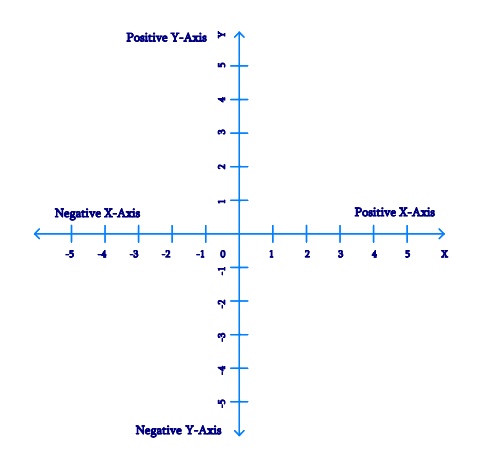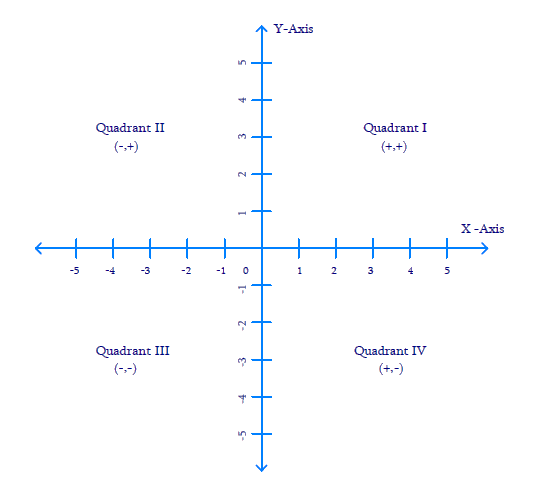# Ex.3.2 Q1 Coordinate Geometry Solution - NCERT Maths Class 9

Go back to  'Ex.3.2'

## Question

Write the answer of each of the following questions:

(i) What is the name of horizontal and the vertical lines drawn to determine the position of any point in the Cartesian plane?

(ii) What is the name of each part of the plane formed by these two lines?

(iii) Write the name of the point where these two lines intersect.

Video Solution
Coordinate Geometry
Ex 3.2 | Question 1

## Text Solution

Steps:

(i) The horizontal line that is drawn to determine the position of any point in the Cartesian plane is called as $$x-$$axis. The vertical line that is drawn to determine the position of any point in the Cartesian plane is called as $$y-$$axis.(ii) The name of each part of the plane that is formed by x-axis and y-axis is called as quadrant.(iii) The point, where the $$x-$$axis and the $$y-$$axis intersect is called as origin $$(O).$$

Video Solution
Coordinate Geometry
Ex 3.2 | Question 1

Learn from the best math teachers and top your exams

• Live one on one classroom and doubt clearing
• Practice worksheets in and after class for conceptual clarity
• Personalized curriculum to keep up with school